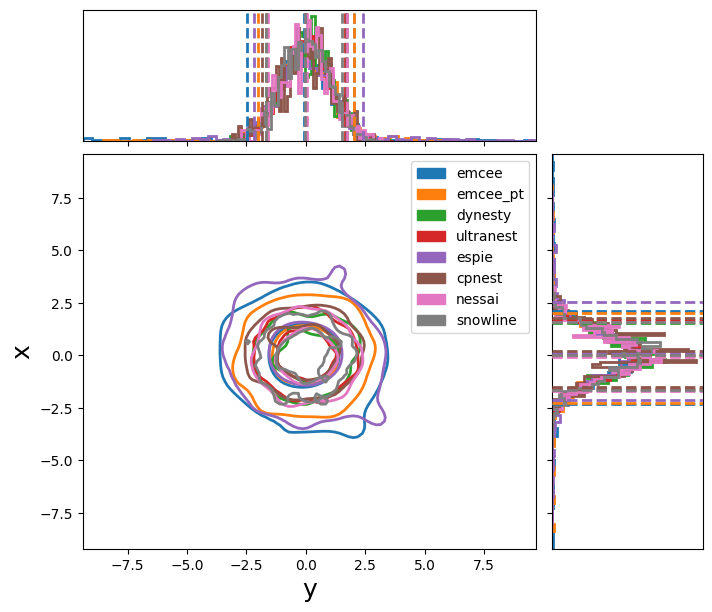# Trying out different samplers¶

This page shows basic configurations for some of the samplers that PyCBC Inference supports. Note that these samplers often have many additional options which you can learn apart from their respective library documentation pages. There very likely are more optimal settings than the example ones here for your problem as these are configured to run quickly.

We’ll use a very simple analytic model to test each sampler. The following is the configuration to set up a unimodal gaussian likelihood. We’ll have each sampler try to fit this.

```[model]
name = test_normal

[variable_params]
x =

[prior-x]
name = uniform
min-x = -10
max-x = 10
```

Each sampler needs nees a slightly different configuration. Below are basic configurations which can analyze this simple problem.

## Emcee¶

```[sampler]
name = emcee
nwalkers = 100
niterations = 200

;##### Other possible options
;effective-nsamples = 1000
;max-samples-per-chain = 1000
;checkpoint-interval = 2000

;[sampler-burn_in]
;burn-in-test = nacl & max_posterior
```

## Parallel-tempered Emcee¶

```[sampler]
name = emcee_pt
nwalkers = 100
ntemps = 4
niterations = 100

;##### Other possible options
;effective-nsamples = 1000
;max-samples-per-chain = 1000
;checkpoint-interval = 2000

;[sampler-burn_in]
;burn-in-test = nacl & max_posterior
```

## PTEmcee¶

```[sampler]
name = ptemcee
nwalkers = 100
ntemps = 4
niterations = 100

;##### Other possible options
;effective-nsamples = 1000
;max-samples-per-chain = 1000
;checkpoint-interval = 2000

;[sampler-burn_in]
;burn-in-test = nacl & max_posterior
```

## Dynesty¶

```[sampler]
name = dynesty
dlogz = 0.1
nlive = 150

; Other arguments (see Dynesty package for details).
; bound, bootstrap, enlarge, update_interval, sample
; loglikelihood-function = loglr
```

## Ultranest¶

```[sampler]
name = ultranest
dlogz = 0.1

;##### Other possible options (see ultranest package for useage)
; update_interval_iter_fraction, update_interval_ncall
; log_interval, show_status, dKL, frac_remain,
; Lepsilon, min_ess, max_iters, max_ncalls,
; max_num_improvement_loops, min_num_live_points,
; cluster_num_live_points
```

## Epsie¶

```[sampler]
name = epsie
nchains = 100
niterations = 100
ntemps = 4

;##### Other possible options
;effective-nsamples = 1000
;max-samples-per-chain = 1000
;checkpoint-interval = 2000

;[sampler-burn_in]
;burn-in-test = nacl & max_posterior

[jump_proposal-x]
name = normal
```

The following are also supported, but require either python3 support only (cpnest) or an external package (multinest).

## cpnest¶

```[sampler]
name = cpnest
nlive = 100
maxmcmc = 1000
verbose = 1

;##### Other possible options
;verbose = True
;loglikelihood-function =
```

## Multinest¶

```[sampler]
name = multinest
nlivepoints = 100

;##### Optional arguments
;evidence-tolerance = 0.1
;sampling-efficiency = 0.3
;checkpoint-interval = 5000
;importance-nested-sampling = True
```

If we run these samplers, we create the following plot: